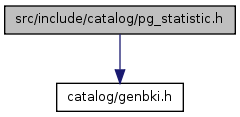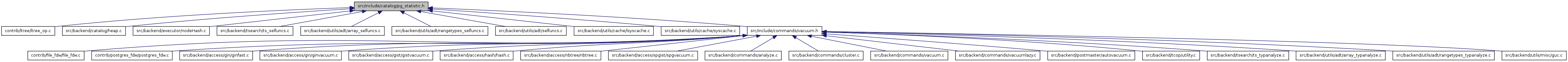# pg_statistic.h File Reference

`#include "catalog/genbki.h"`
Include dependency graph for pg_statistic.h:This graph shows which files directly or indirectly include this file:Go to the source code of this file.

## Defines

#define StatisticRelationId   2619
#define STATISTIC_NUM_SLOTS   5
#define Natts_pg_statistic   26
#define Anum_pg_statistic_starelid   1
#define Anum_pg_statistic_staattnum   2
#define Anum_pg_statistic_stainherit   3
#define Anum_pg_statistic_stanullfrac   4
#define Anum_pg_statistic_stawidth   5
#define Anum_pg_statistic_stakind1   7
#define Anum_pg_statistic_stakind2   8
#define Anum_pg_statistic_stakind3   9
#define Anum_pg_statistic_stakind4   10
#define Anum_pg_statistic_stakind5   11
#define Anum_pg_statistic_staop1   12
#define Anum_pg_statistic_staop2   13
#define Anum_pg_statistic_staop3   14
#define Anum_pg_statistic_staop4   15
#define Anum_pg_statistic_staop5   16
#define Anum_pg_statistic_stanumbers1   17
#define Anum_pg_statistic_stanumbers2   18
#define Anum_pg_statistic_stanumbers3   19
#define Anum_pg_statistic_stanumbers4   20
#define Anum_pg_statistic_stanumbers5   21
#define Anum_pg_statistic_stavalues1   22
#define Anum_pg_statistic_stavalues2   23
#define Anum_pg_statistic_stavalues3   24
#define Anum_pg_statistic_stavalues4   25
#define Anum_pg_statistic_stavalues5   26
#define STATISTIC_KIND_MCV   1
#define STATISTIC_KIND_HISTOGRAM   2
#define STATISTIC_KIND_CORRELATION   3
#define STATISTIC_KIND_MCELEM   4
#define STATISTIC_KIND_DECHIST   5
#define STATISTIC_KIND_RANGE_LENGTH_HISTOGRAM   6
#define STATISTIC_KIND_BOUNDS_HISTOGRAM   7

## Typedefs

typedef FormData_pg_statisticForm_pg_statistic

## Functions

CATALOG (pg_statistic, 2619) BKI_WITHOUT_OIDS

## Variables

FormData_pg_statistic

## Define Documentation

 #define Anum_pg_statistic_staattnum   2

Definition at line 136 of file pg_statistic.h.

Referenced by RemoveStatistics(), and update_attstats().

Definition at line 140 of file pg_statistic.h.

Referenced by update_attstats().

 #define Anum_pg_statistic_stainherit   3

Definition at line 137 of file pg_statistic.h.

Referenced by update_attstats().

 #define Anum_pg_statistic_stakind1   7

Definition at line 141 of file pg_statistic.h.

Referenced by update_attstats().

 #define Anum_pg_statistic_stakind2   8

Definition at line 142 of file pg_statistic.h.

 #define Anum_pg_statistic_stakind3   9

Definition at line 143 of file pg_statistic.h.

 #define Anum_pg_statistic_stakind4   10

Definition at line 144 of file pg_statistic.h.

 #define Anum_pg_statistic_stakind5   11

Definition at line 145 of file pg_statistic.h.

 #define Anum_pg_statistic_stanullfrac   4

Definition at line 138 of file pg_statistic.h.

Referenced by update_attstats().

 #define Anum_pg_statistic_stanumbers1   17

Definition at line 151 of file pg_statistic.h.

Referenced by get_attstatsslot(), and update_attstats().

 #define Anum_pg_statistic_stanumbers2   18

Definition at line 152 of file pg_statistic.h.

 #define Anum_pg_statistic_stanumbers3   19

Definition at line 153 of file pg_statistic.h.

 #define Anum_pg_statistic_stanumbers4   20

Definition at line 154 of file pg_statistic.h.

 #define Anum_pg_statistic_stanumbers5   21

Definition at line 155 of file pg_statistic.h.

 #define Anum_pg_statistic_staop1   12

Definition at line 146 of file pg_statistic.h.

Referenced by update_attstats().

 #define Anum_pg_statistic_staop2   13

Definition at line 147 of file pg_statistic.h.

 #define Anum_pg_statistic_staop3   14

Definition at line 148 of file pg_statistic.h.

 #define Anum_pg_statistic_staop4   15

Definition at line 149 of file pg_statistic.h.

 #define Anum_pg_statistic_staop5   16

Definition at line 150 of file pg_statistic.h.

 #define Anum_pg_statistic_starelid   1

Definition at line 135 of file pg_statistic.h.

Referenced by RemoveStatistics(), and update_attstats().

 #define Anum_pg_statistic_stavalues1   22

Definition at line 156 of file pg_statistic.h.

Referenced by get_attstatsslot(), and update_attstats().

 #define Anum_pg_statistic_stavalues2   23

Definition at line 157 of file pg_statistic.h.

 #define Anum_pg_statistic_stavalues3   24

Definition at line 158 of file pg_statistic.h.

 #define Anum_pg_statistic_stavalues4   25

Definition at line 159 of file pg_statistic.h.

 #define Anum_pg_statistic_stavalues5   26

Definition at line 160 of file pg_statistic.h.

 #define Anum_pg_statistic_stawidth   5

Definition at line 139 of file pg_statistic.h.

Referenced by update_attstats().

 #define Natts_pg_statistic   26

Definition at line 134 of file pg_statistic.h.

 #define STATISTIC_KIND_BOUNDS_HISTOGRAM   7

Definition at line 291 of file pg_statistic.h.

Referenced by calc_hist_selectivity().

 #define STATISTIC_KIND_CORRELATION   3

Definition at line 232 of file pg_statistic.h.

Referenced by btcostestimate().

 #define STATISTIC_KIND_DECHIST   5

Definition at line 269 of file pg_statistic.h.

Referenced by calc_arraycontsel(), and scalararraysel_containment().

 #define STATISTIC_KIND_HISTOGRAM   2
 #define STATISTIC_KIND_MCELEM   4

Definition at line 256 of file pg_statistic.h.

Referenced by calc_arraycontsel(), scalararraysel_containment(), and tsquerysel().

 #define STATISTIC_KIND_MCV   1

Definition at line 203 of file pg_statistic.h.

 #define STATISTIC_KIND_RANGE_LENGTH_HISTOGRAM   6

Definition at line 280 of file pg_statistic.h.

Referenced by calc_hist_selectivity(), and calc_rangesel().

 #define STATISTIC_NUM_SLOTS   5

Definition at line 120 of file pg_statistic.h.

Referenced by compute_array_stats().

 #define StatisticRelationId   2619

## Typedef Documentation

 typedef FormData_pg_statistic* Form_pg_statistic

Definition at line 128 of file pg_statistic.h.

## Function Documentation

 CATALOG ( pg_statistic , 2619 )

Definition at line 31 of file pg_statistic.h.

```{
/* These fields form the unique key for the entry: */
Oid         starelid;       /* relation containing attribute */
int16       staattnum;      /* attribute (column) stats are for */
bool        stainherit;     /* true if inheritance children are included */

/* the fraction of the column's entries that are NULL: */
float4      stanullfrac;

/*
* stawidth is the average width in bytes of non-null entries.  For
* fixed-width datatypes this is of course the same as the typlen, but for
* var-width types it is more useful.  Note that this is the average width
* of the data as actually stored, post-TOASTing (eg, for a
* moved-out-of-line value, only the size of the pointer object is
* counted).  This is the appropriate definition for the primary use of
* the statistic, which is to estimate sizes of in-memory hash tables of
* tuples.
*/
int32       stawidth;

/* ----------------
* stadistinct indicates the (approximate) number of distinct non-null
* data values in the column.  The interpretation is:
*      0       unknown or not computed
*      > 0     actual number of distinct values
*      < 0     negative of multiplier for number of rows
* The special negative case allows us to cope with columns that are
* unique (stadistinct = -1) or nearly so (for example, a column in
* which values appear about twice on the average could be represented
* by stadistinct = -0.5).  Because the number-of-rows statistic in
* pg_class may be updated more frequently than pg_statistic is, it's
* important to be able to describe such situations as a multiple of
* the number of rows, rather than a fixed number of distinct values.
* But in other cases a fixed number is correct (eg, a boolean column).
* ----------------
*/

/* ----------------
* To allow keeping statistics on different kinds of datatypes,
* we do not hard-wire any particular meaning for the remaining
* statistical fields.  Instead, we provide several "slots" in which
* statistical data can be placed.  Each slot includes:
*      kind            integer code identifying kind of data (see below)
*      op              OID of associated operator, if needed
*      numbers         float4 array (for statistical values)
*      values          anyarray (for representations of data values)
* The ID and operator fields are never NULL; they are zeroes in an
* unused slot.  The numbers and values fields are NULL in an unused
* slot, and might also be NULL in a used slot if the slot kind has
* no need for one or the other.
* ----------------
*/

int16       stakind1;
int16       stakind2;
int16       stakind3;
int16       stakind4;
int16       stakind5;

Oid         staop1;
Oid         staop2;
Oid         staop3;
Oid         staop4;
Oid         staop5;

#ifdef CATALOG_VARLEN           /* variable-length fields start here */
float4      stanumbers1;
float4      stanumbers2;
float4      stanumbers3;
float4      stanumbers4;
float4      stanumbers5;

/*
* Values in these arrays are values of the column's data type, or of some
* related type such as an array element type.  We presently have to cheat
* quite a bit to allow polymorphic arrays of this kind, but perhaps
* someday it'll be a less bogus facility.
*/
anyarray    stavalues1;
anyarray    stavalues2;
anyarray    stavalues3;
anyarray    stavalues4;
anyarray    stavalues5;
#endif
} FormData_pg_statistic;
```

## Variable Documentation

Definition at line 118 of file pg_statistic.h.